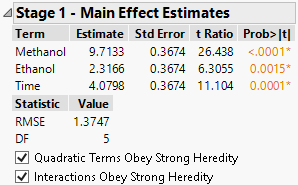Publication date: 05/24/2021

## Stage 1 - Main Effect Estimates

The Main Effect Estimates report lists main effects that are identified as active. Main effects with p-values less than the threshold p-value are considered active.

If fake factors or center point replicates are available, an estimator of error variance that is independent of the model is constructed. The main effects are tested against this estimate.

If no fake factors or center point replicates are available, subsets of main effects are tested sequentially against an estimate of error variance constructed from the inactive main effects. For this procedure to be viable, at least one of the main effects must be inactive.

In either case, variability from the inactive main effects is pooled into the error variance used to test the main effects.

Figure 8.8 Stage 1 ReportTerm

Main effects identified as active. These effects have p-values less than the threshold when tested as described in Stage 1 Methodology.

Estimate

Parameter estimate for a regression fit of Y on the main effects.

Std Error

The standard error of the estimate, computed using the Stage 1 RMSE.

t Ratio

The Estimate divided by its Std Error.

Prob>|t|

The p-value computed using the t Ratio and the degrees of freedom for error (DF).

RMSE

The square root of the mean square error that results from the Stage 1 analysis.

If fake factors or centerpoint replicates are available, the mean square error is the estimate of variance from fake factors and centerpoints pooled with the variance estimate constructed from the main effects that are not identified as active.

If no fake factors or centerpoint replicates are available, the mean square error is the estimate of variance constructed from the main effects that are not identified as active.

DF

The degrees of freedom associated with the error estimate used to construct RMSE.

If fake factors or centerpoint replicates are available, DF is the sum of the number of fake factors, centerpoint replicates, and main effects not identified as active.

If no fake factors or centerpoint replicates are available, DF is the number of main effects that are not identified as active.